##### Santiago Morante Cendrero1 November, 2018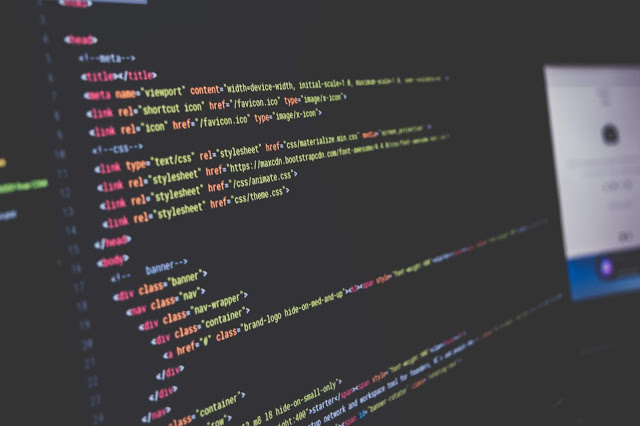For many machine learning algorithms, normalizing data for analysis is a must. A supervised example would be neural networks. It is known that normalizing the data being input to the networks improves the results. If you don’t believe me it’s OK (no offense taken), but you may prefer to believe Yann Le Cunn (Director of AI Research in Facebook and founding father of convolution networks) by checking section 4.3 of this paper.

Convergence [of backdrop] is usually faster if the average of each input variable over the training set is close to zero. Among others, one reason is that when the neural network tries to correct the error performed in a prediction, it updates the network by an amount proportional to the input vector, which is bad if input is large
.

Another example in this case of an unsupervised algorithm, is K-means. This algorithm tries to group data in clusters so that the data in each cluster shares some common characteristics. This algorithm performs two steps:

• Assign centers of clusters in some point in space (random at first try, calculating the centroid of each cluster the rest of the time)
• Associate each point to the closest center.
In this second step, the distances between each point and the centers are calculated usually as a Minkowski distance (commonly the famous Euclidean distance). Each feature weights the same in the calculation, so features measured in high ranges will influence more than those measured in low ranges e.g. the same feature would have more influence in the calculation if measured in millimeters than in kilometers (because the numbers would be bigger). So the scale of the features must be in a comparable range.
Now you know that normalization is important, let´s see what options we have to normalize our data.

## A couple of ways to normalize data:

### Feature scaling

Each feature is normalized within its limits.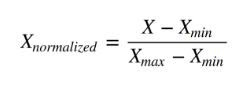Figure 1, normalization formula
This is a common technique used to scale data into a range. But the problem when normalizing each feature within its empirical limits (so that the maximum and the minimum are found in this column) is that noise may be amplified.
One example: imagine we have Internet data from a particular house and we want to make a model to predict something (maybe the price to charge). One of our hypothetical features could be the bandwidth of the fiber optic connection. Suppose the house purchased a 30Mbit Internet connection, so the bit rate is approximately the same every time we measure it (lucky guy).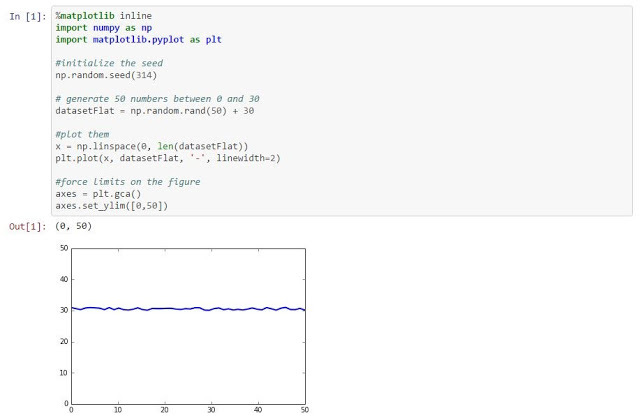Figure 2, Connection speed over 50 days
It looks like a pretty stable connection right? As the bandwidth is measured in a scale far from 1, let us scale it between 0 and 1 using our feature scaling method (sklearn.preprocessing.MinMaxScaler).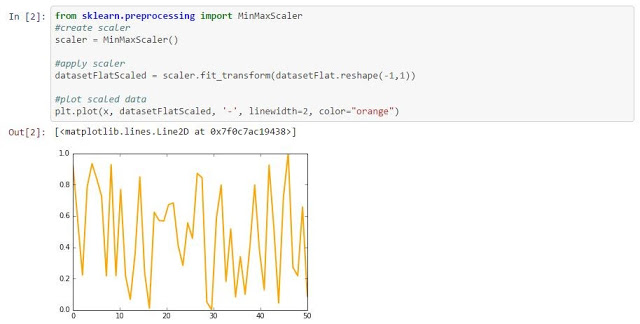Figure 3, Connection speed / day in scale 0-1.
After the scaling, our data is distorted. What was an almost flat signal, now looks like a connection with a lot of variation. This tells us that feature scaling is not adequate to nearly constant signals.

### Standard scaler

Next try. OK, scaling in a range didn’t work for a noisy flat signal, but what about standardizing the signal? Each feature would be normalized by: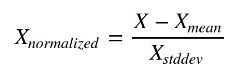Figure 4, Standard scaling formula
This could work on the previous case, but don’t open the champagne yet. Mean and standard deviation are very sensitive to outliers (small demonstration). This means that outliers may attenuate the non-outlier part of the data.
Now imagine we have data about how often the word “hangover” is posted on Facebook (for real). The frequency is like a sine wave, with lows during the weekdays and highs on weekends. It also has big outliers after “Halloween” and similar dates. We have idealized this situation with the next data set (3 parties in 50 days. Not bad).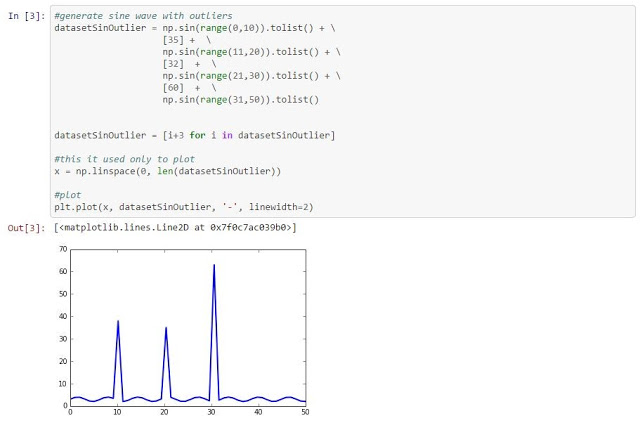Figure 5, Number of times the word “hangover” is used in Facebook / days.
Despite having outliers, we would like to be able to distinguish clearly that there is a measurable difference between weekdays and weekends. Now we want to predict something (that’s our business) and we would like to preserve the fact that during the weekends the values are higher, so we think of standardizing the data (sklearn.preprocessing.StandardScaler). We check the basic parameters of standardization.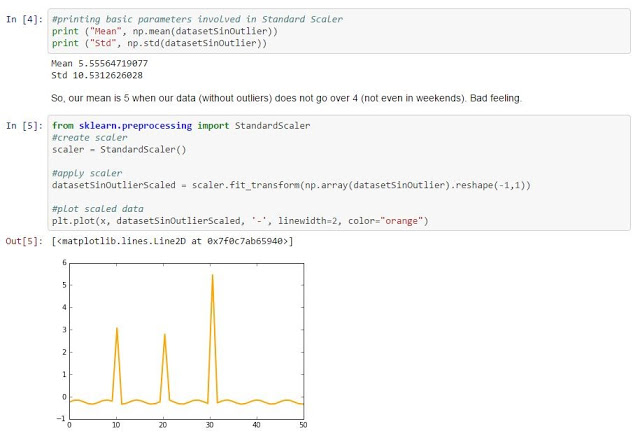Figure 6, Standard standardization for the above data is not a good choice.

What happened? First, we were not able to scale the data between 0 and 1. Second, we now have negative numbers, which is not a dead end, but complicates the analysis. And third, now we are unable to clearly distinguish the differences between weekdays and weekends (all close to 0), because outliers have interfered with the data.

From a very promising data, we now have an almost irrelevant one. One solution to this situation could be to pre-process the data and eliminate the outliers (things change with outliers).

### Scaling over the maximum value

The next idea that comes to mind is to scale the data by dividing it by its maximum value. Let´s see how it behaves with our data sets (sklearn.preprocessing.MaxAbsScaler).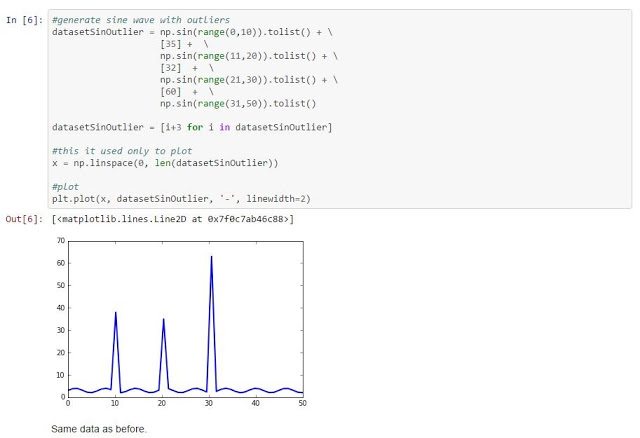Figure 7, data divided by maximum value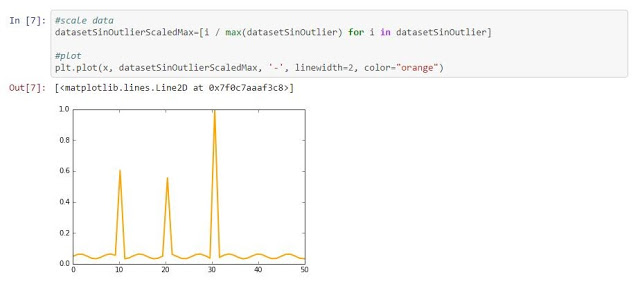Figure 8, data scaled over the maximum

Good! Our data is in range 0,1… But, wait. What happened with the differences between weekdays and weekends? They are all close to zero! As in the case of standardization, outliers flatten the differences among the data when scaling over the maximum.

### Normalizer

The next tool in the box of the data scientist is to normalize samples individually to unit norm (check this if you don’t remember what a norm is).Figure 9, samples individually sampled to unit norm

This data rings a bell in your head right? Let’s normalize it (here by hand, but also available as sklearn.preprocessing.Normalizer).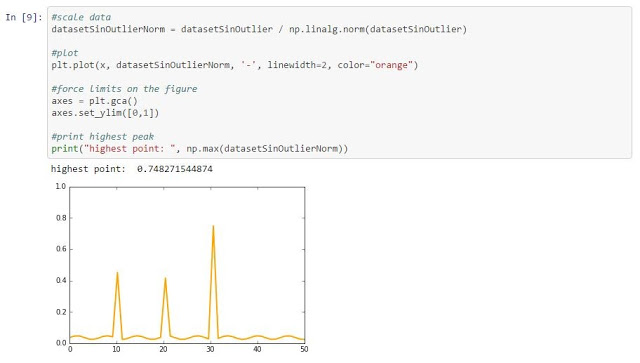Figure 10, the data was then normalized

At this point in the post, you know the story, but this case is worse than the previous one. In this case we don’t even get the highest outlier as 1, it is scaled to 0.74, which flattens the rest of the data even more.

### Robust scaler

The last option we are going to evaluate is Robust scaler. This method removes the median and scales the data according to the Interquartile Range (IQR). It is supposed to be robust to outliers.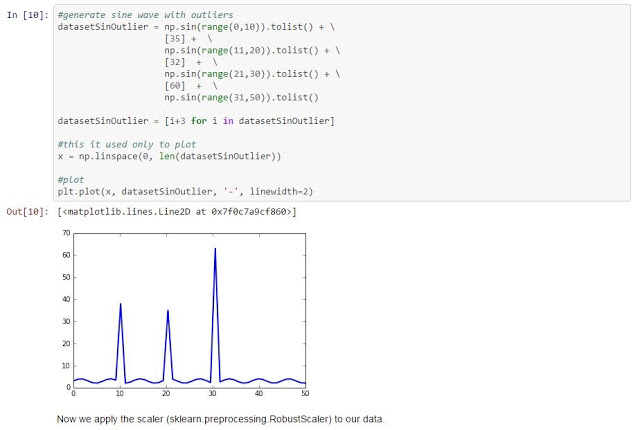Figure 11, the median data removed and scaled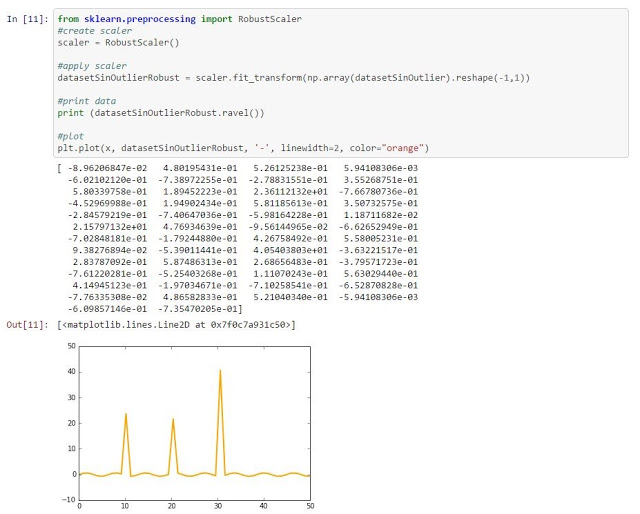Figure 12, use of Robust scaler

You may not see it in the plot (but you can see it in the output), but this scaler introduced negative numbers and did not limit the data to the range [0, 1]. (OK, I quit).

There are others methods to normalize your data (based on PCA, taking into account possible physical boundaries, etc), but now you know how to evaluate whether your algorithm is going to influence your data negatively.

### Things to remember (basically, know your data):

Normalization may (possibly [dangerously]) distort your data. There is no ideal method to normalize or scale all the data sets. Thus it is the job of the data scientist to know how the data is distributed, know the existence of outliers, check ranges, know the physical limits (if any) and so on. With this knowledge, one can select the best technique to normalize the feature, probably using a different method for each feature.

If you know nothing about your data, I would recommend you to first check the existence of outliers (remove them if necessary) and then scale over the maximum of each feature (while crossing your fingers).

Written by Santiago Morante, PhD, Data Scientist at LUCA Consulting Analytics